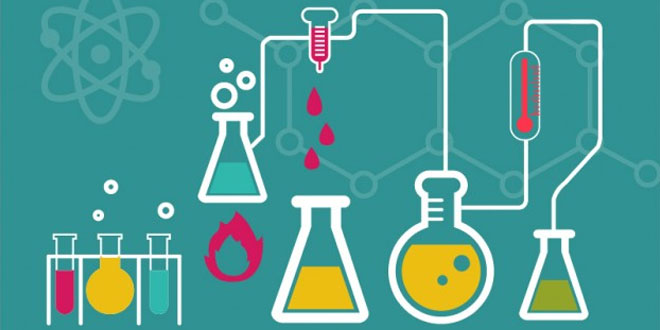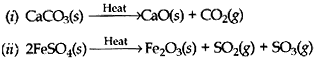Wednesday , September 28 2022# Chemical Reaction & Equations: 10 Science

## Chemical Reaction & Equations: 10 Science – Chapter 1

#### Question: What is a balanced chemical equation? Why should chemical equations be balanced?

Answer: An equation for a chemical reaction is an equation in which the number of atoms for each element in the reaction and the total charge is the same for both the reactants and the products. In other words, the mass and the charge are balanced on both sides of the reaction.

#### Question: Translate the following statements into chemical equations and then balance them. 1. Hydrogen gas combines with nitrogen to form ammonia 2. Hydrogen sulfide gas burns in air to give water and sulfur dioxide 3. Barium chloride reacts with Aluminium sulfate to give Aluminium chloride and a precipitate of barium sulphate 4. Potassium metal reacts with water to give a potassium hydroxide and hydrogen gas 5. Aluminium chloride reacts with ammonium hydroxide to from a gelatinous white precipitate of aluminium hydroxide and salt of ammonium chloride.#### Question: Balance the following chemical equations. (1) HNO3 + Ca(OH)2  → Ca(NO3)2 + H2O (2) NaOH + H2SO4 → Na2SO4 + H2O (3) NaCl+ AgNO3 → AgCl + NaNO3 (4) BaCl2 + H2SO24 → BaSO4 + HCl

1. 2HNO+ Ca(OH)2  → Ca(NO3)2 + 2H2O
2. 2NaOH + H2SO4 → Na2SO+ 2H2O
3. NaCl + AgNO→ AgCl + NaNO3
4. BaCl+ H2SO→ BaSO+ 2HCl

#### Question: Write the balanced chemical equations for the following reactions: (1) Calcium hydroxide + Carbon dioxide → Calcium carbonate + Water (2) Zinc + Silver nitrate → Zinc nitrate + Silver (3) Aluminium + Copper Chloride → Aluminium chloride + Copper (4) Barium chloride + Potassium sulphate → Barium sulphate + Potassium chloride.

1. Ca(OH)2 + CO2 → CaCO3+ H2O
2. Zn + 2AgNO3 → Zn(NO3)2 + 2Ag
3. 2Al + 3CuCl2 → 2AlCl3 + 3Cu
4. BaCl2 + K2SO4 → BaSO4 + 2KCl

#### Question: Write the balanced chemical equation for the following and identify the type of reaction of each case. (1) Potassium bromide (aq) + Barium iodide (aq) → Potassium iodede (aq) + Barium bromide (s) (2) Zinc carbonate (s) → Zinc oxide (s) + Carbon dioxide (g) (3) Hydrogen (g) + Chlorine (g) → Hydrogen chloride (g) (4) Magnesium (s) + Hydrochloric acid (aq) → Magnesium chloride (aq) + Hydrogen (g)

1. 2KBr (aq) + BaI2 (aq) → 2KI (aq) + BaBr2 (aq) — Double Displacement reaction
2. ZnCO3 (s) → ZnO(s) + CO2 (g) — Decomposition reaction
3. H2 (g) + Cl2 (g) → 2HCl (g) — Combination reaction
4. Mg (s) + 2HCl (aq) → MgCl2 (aq) + H2 (g) — Displacement reaction

#### Question: What is meant by exothermic and endothermic reactions? Give examples.

Answer: An exothermic reaction is one that releases heat.

If 1 mole of N2 molecule reacts with 1 mole of O2 molecule, the heat of 184 KJ has to be supplied to initiate the reaction to give 1 mole of NO molecule. This means that the bonds between N – N and O – O are so strong that they do not break easily. N2 has triple covalent bond between the two N atoms. O2 has a double covalent bond. Thus energy has to be put into the reaction to break the strong bonds. Thus the above reaction is a good example of an endothermic reaction.

#### Question: Why is respiration considered an exothermic reaction?

Answer: During respiration, glucose combines with oxygen in the cells of our body to form carbon dioxide and water along with the production of energy.

Glucose + oxygen → carbon dioxide + water + energy

It is an exothermic reaction, because energy is produced during this process.

#### Question: Why are decomposition reactions called the opposite of combination reactions? Write equations for decomposition reactions.

Answer: Essentially, decomposition reactions are the opposite of combination reactions. A compound decomposes (i.e., “splits-up”) into two or more compounds and/or elements. For example, mercury (II) oxide will, decompose into mercury metal and oxygen, upon heating:

2HgO → 2Hg + O2

Since heat had to be added to make this reaction occur, it is an endothermic reaction. Most decomposition reactions are endothermic. Another example of decomposition reaction is the heating of calcium carbonate (sea shells, chalk):#### Question: What is the difference between displacement and double displacement reactions? Write relevant equations for the above?

Answer: The difference between the displacement and double displacement reactions is that in a displacement reaction one element displaces another by virtue of it being more reactive whereas in a double displacement two anions and two cations switch places between two compounds respectively.

For example:

CuSO4 (aq) + Zn(s) → ZnSO4 + Cu(s)

Blue copper sulphate solution reacting with solid zinc will give rise to colorless zinc sulphate solution and solid copper. Thus Zn displaces Cu in the salt form. Zn is more reactive than Cu.

An example of double displacement reaction is the reaction between sodium carbonate and calcium chloride, both in aqueous

#### Question: In the refining of silver, the recovery of silver from silver nitrate solution involves displacement by copper metal. Write down the reaction involved.

Cu(s) + 2AgNO3 (aq) → Cu(NO3)2 (aq) + 2Ag(s)

#### Question: What do you mean by a precipitation reaction? Explain by giving examples:

A precipitation reaction is a reaction in which soluble ions in separate solutions are mixed together to form an insoluble compound that settles out of solution as a solid. This insoluble compound is called a precipitate.

An example of a precipitation reaction

Aqueous silver nitrate (AgNO3), when added to a solution containing potassium chloride (KCl), precipitates a white solid, and silver chloride is observed.

AgNO3 (aq) + KCl(aq) → AgCl(s) + KNO3(aq)

The silver chloride (AgCl) has formed a solid, which is observed as a precipitate.

#### Question: A shiny brown colored element ‘X’ on heating in the air becomes black in colour. Name the element ‘X’ and the black colored compound formed.

Answer: The shiny brown colored element X is copper metal (Cu). When copper metal is heated in air, it forms a black colored compound copper oxide. So, the black colored compound is the copper oxide or copper (II) oxide, CuO.

#### Question: Why do we apply paint on iron articles?

Answer: Rust is a soft and porous substance, which gradually falls from the surface of an iron object, and then the iron below starts rusting. Thus, rusting of iron (or corrosion of iron) is a continuous process which, if not prevented in time, eats up the whole iron object. So, when we apply paint on iron articles it reduces the rusting of iron.

#### Question: Oil and fat containing food items are flushed with nitrogen. Why?

Answer: Packaging fat and oil containing foods in nitrogen gas can prevent rancidity. When the fat and oil present in food materials gets oxidized (in air), their oxidation products have an unpleasant smell and taste. When it is surrounded by unreactive gas, nitrogen, there is no oxygen (of air) to cause its oxidation and make it rancid.

## Sources of Energy: 10th Science Chapter 14

Class: 10th Class Subject: Science Chapter: Chapter 14: Sources of Energy Quiz: – Questions MCQs: …